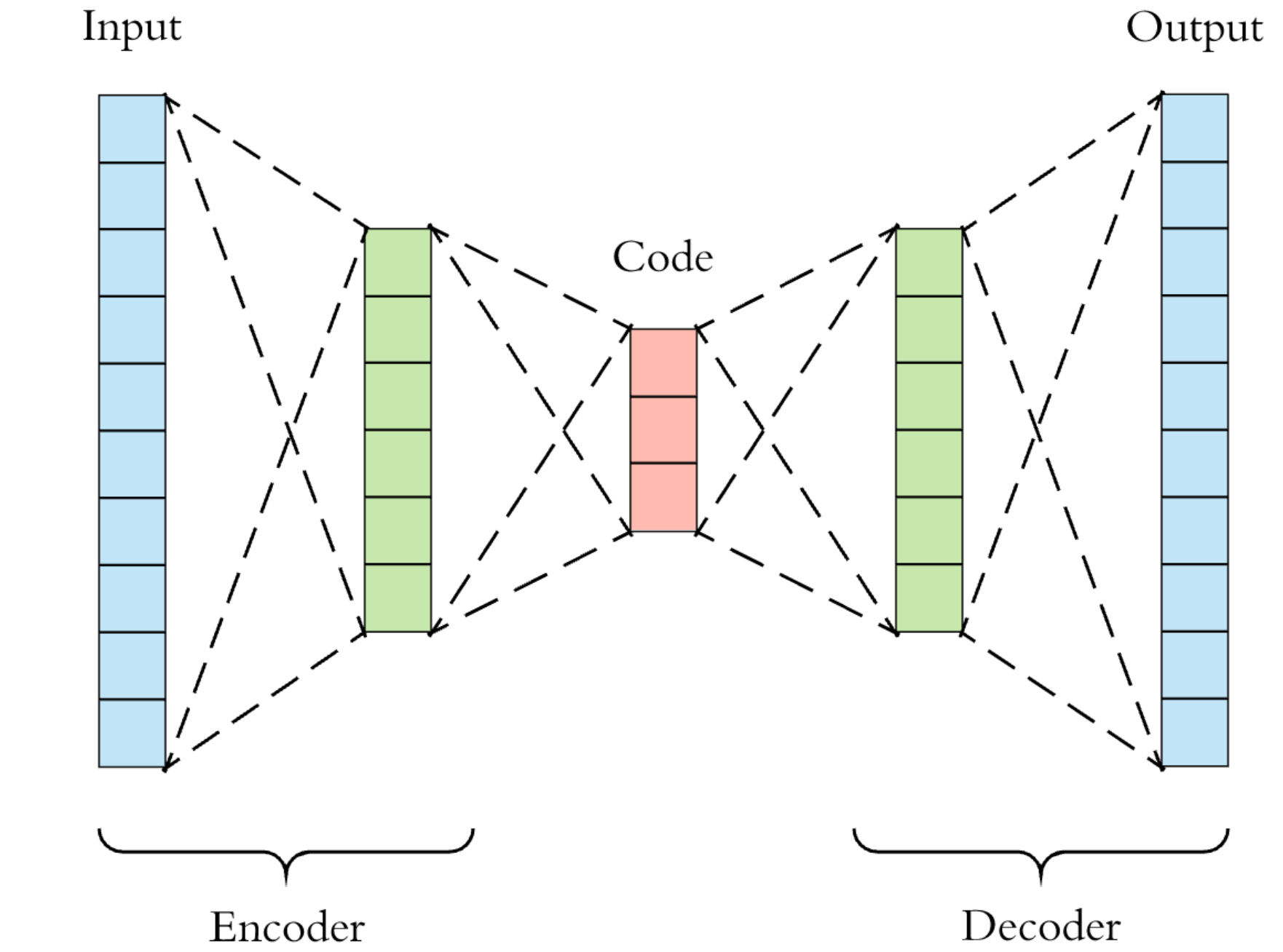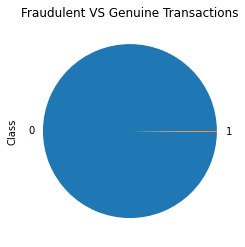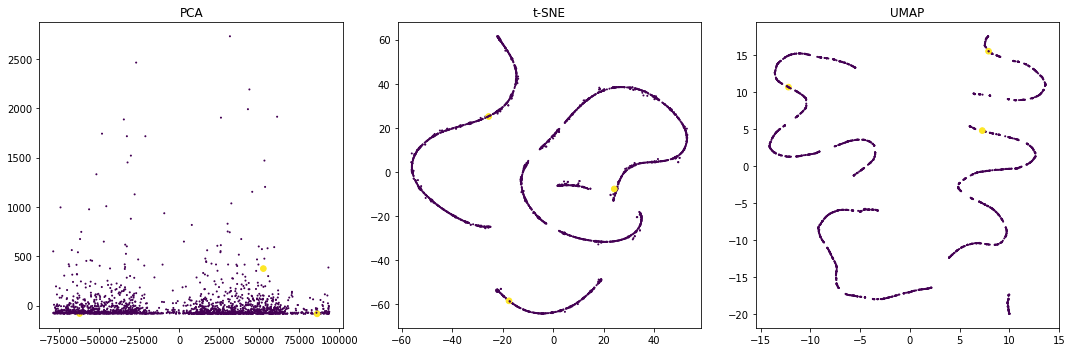# Semi-Supervised Fraud Detection

Kaggle kernel

## Intro¶

dataset: Credit Card Fraud Detection

keywords: fraud detection, novelty detection, ensembling, learning representation, semi-supervised learning

Usually, datasets for fraud detection are highly unbalanced. Instead of trying to resample the dataset, we are going to approach this problem as a Novelty Detection problem.

In this kernel, we will describe the dataset briefly and then we will compare multiple machine learning algorithms using some evaluation metrics.

Most common machine learning algorithms for this kind of tasks are the following:

1. Isolation forest
2. One class SVM
3. Autoencoders

## Isolation Forest¶source

The Isolation Forest algorithm isolates observations by randomly selecting a feature and then randomly selecting a split value between the maximum and minimum values of the selected feature. The logic argument goes: isolating anomaly observations is easier because only a few conditions are needed to separate those cases from the normal observations. On the other hand, isolating normal observations require more conditions. Therefore, an anomaly score can be calculated as the number of conditions required to separate a given observation.

## One Class SVM (OCSVM)¶source

A One-Class Support Vector Machine is an unsupervised learning algorithm that is trained only on the ‘normal’ data, in our case the negative examples. It learns the boundaries of these points and is therefore able to classify any points that lie outside the boundary as, you guessed it, outliers.

## Autoencoders¶

source

An autoencoder is a neural network that is trained to attempt to copy its input to its output. Internally, it has a hidden layer h that describes a code used to represent the input. The network may be viewed as consisting of two parts: an encoder function h = f (x) and a decoder that produces a reconstruction r = g(h).In our case, we will train the autoencoder using samples of normal transactions only. When we feed the autoencoder an anomaly sample, the representation should have larger loss error. Therefore, by defining a loss threashold, this ANN model will work as novelty detection model.

## Implementation¶In :
import numpy as np
import pandas as pd
from sklearn.ensemble import IsolationForest
from sklearn.svm import OneClassSVM
from sklearn.preprocessing import StandardScaler
from sklearn.model_selection import train_test_split
from sklearn.metrics import confusion_matrix, recall_score, f1_score, accuracy_score, precision_score
from sklearn.ensemble import VotingClassifier, RandomForestClassifier
from tqdm.notebook import tqdm, trange
from typing import NoReturn, Union, List
from mlxtend.classifier import EnsembleVoteClassifier
import matplotlib.pyplot as plt
import seaborn as sns
import tensorflow as tf
from umap import UMAP

In :
df = pd.read_csv('../input/creditcardfraud/creditcard.csv')

Out:
Time V1 V2 V3 V4 V5 V6 V7 V8 V9 ... V21 V22 V23 V24 V25 V26 V27 V28 Amount Class
0 0.0 -1.359807 -0.072781 2.536347 1.378155 -0.338321 0.462388 0.239599 0.098698 0.363787 ... -0.018307 0.277838 -0.110474 0.066928 0.128539 -0.189115 0.133558 -0.021053 149.62 0
1 0.0 1.191857 0.266151 0.166480 0.448154 0.060018 -0.082361 -0.078803 0.085102 -0.255425 ... -0.225775 -0.638672 0.101288 -0.339846 0.167170 0.125895 -0.008983 0.014724 2.69 0
2 1.0 -1.358354 -1.340163 1.773209 0.379780 -0.503198 1.800499 0.791461 0.247676 -1.514654 ... 0.247998 0.771679 0.909412 -0.689281 -0.327642 -0.139097 -0.055353 -0.059752 378.66 0
3 1.0 -0.966272 -0.185226 1.792993 -0.863291 -0.010309 1.247203 0.237609 0.377436 -1.387024 ... -0.108300 0.005274 -0.190321 -1.175575 0.647376 -0.221929 0.062723 0.061458 123.50 0
4 2.0 -1.158233 0.877737 1.548718 0.403034 -0.407193 0.095921 0.592941 -0.270533 0.817739 ... -0.009431 0.798278 -0.137458 0.141267 -0.206010 0.502292 0.219422 0.215153 69.99 0

5 rows × 31 columns

## Dataset¶

• V{1-28}: PCA decompossition outcome
• Class label (0: normal, 1: fraudulent)
• Time: Number of seconds elapsed between this transaction and the first transaction in the dataset
• Amount: transaction amount
In :
# use a dataset sample for development purposes
dev = False

In :
df.groupby(['Class']).Class.count().plot(kind='pie', title='Fraudulent VS Genuine Transactions')

Out:
<matplotlib.axes._subplots.AxesSubplot at 0x7fa23c5ab6d8>In :
df_s = df.sample(2000)
X = df_s[[_ for _ in df.columns if _ != 'Class']]
from sklearn.decomposition import PCA, IncrementalPCA, LatentDirichletAllocation
from sklearn.manifold import TSNE
pca = PCA(n_components=2)
tsne = TSNE(n_components=2)
ump = UMAP(n_components=2)
ipca = IncrementalPCA(n_components=2)
x_pca = pca.fit_transform(X)
x_tsne = tsne.fit_transform(X)
x_umap = ump.fit_transform(X)


PCA and t-SNE will be used for further dimensionality reduction in order to visualize a sample of the dataset

In :
fig, axes = plt.subplots(nrows=1, ncols=3, figsize=(15, 5))
sizes = pd.Series(df_s['Class']+1).pow(5) # represent fraud with bigger point
axes.scatter(x_pca[:, 0], x_pca[:, 1], s=sizes, c=df_s['Class'].values)
axes.scatter(x_tsne[:, 0], x_tsne[:, 1], s=sizes, c=df_s['Class'].values)
axes.scatter(x_umap[:, 0], x_umap[:, 1], s=sizes, c=df_s['Class'].values)
axes.set_title('PCA')
axes.set_title('t-SNE')
axes.set_title('UMAP')
fig.tight_layout()
plt.show()# Preprocessing¶

Time and Amount fields should be scaled

During this step, the dataset will be splited in two parts:

1. ~283K samples of normat transactions (Training set)
2. All fraudulent samples and equal number of normal samples (Test set)

Novelty detection is concered as semi-supervised task due to the fact that only the normal samples are used during the phase of training. During the phase of evaluation, a balanced subset of normal and fraudulent samples will be used.

In :
# time and amount scaling
df['Time'] = StandardScaler().fit_transform(df['Time'].values.reshape(-1, 1))
df['Amount'] = StandardScaler().fit_transform(df['Amount'].values.reshape(-1, 1))

df_anom = df[df['Class'] == 1]
df_norm = df[df['Class'] == 0]
if dev:
df_norm = df_norm.sample(5000, random_state=42)
df_test_norm = df_norm.sample(df_anom.shape)
df_test = pd.concat([
df_anom,
df_test_norm
])
df_train = df_norm.drop(df_test_norm.index)

feature_cols = [_ for _ in df.columns if _ != 'Class']

In :
X_train = df_train[feature_cols]
y_train = df_train['Class'] # will not be used
X_test = df_test[feature_cols]
y_test = df_test['Class'] # for evaluation
print('''
train: [{:>8} x {:<5}]
test: [{:>8} x {:<5}]
'''.format(*X_train.shape, *X_test.shape))

train: [  283823 x 30   ]
test: [     984 x 30   ]


In :
def sensitivity_keras(y_true, y_pred):
"""
credits: https://datascience.stackexchange.com/a/40746/28592

param:
y_pred - Predicted labels
y_true - True labels
Returns:
Specificity score
"""
neg_y_true = 1 - y_true
neg_y_pred = 1 - y_pred
fp = tf.keras.backend.sum(neg_y_true * y_pred)
tn = tf.keras.backend.sum(neg_y_true * neg_y_pred)
specificity = tn / (tn + fp + tf.keras.backend.epsilon())
return specificity


# Training¶

We are going to define some wrapper, these classes will work as adapters in order to have a uniform prediction.

In :
class Scaled_IsolationForest(IsolationForest):
"""
The purpose of this class is to transform prediction values from {-1, 1} to {1,0}
"""

def predict(self, X):
pred = super().predict(X)
scale_func = np.vectorize(lambda x: 1 if x == -1 else 0)
return scale_func(pred)

class Scaled_OneClass_SVM(OneClassSVM):
"""
Transform OneClassSVM prediction into 0s and 1s
"""
def predict(self, X):
return np.array([y==-1 for y in super().predict(X)])

class NoveltyDetection_Sequential(tf.keras.models.Sequential):
"""
This custom tf.keras.models.Sequential based class transforms autoencoder's output into {1,0}.
Output value is determined based on reproduction (decode) loss. If reproduction loss is more than a threashold then, the inpu sample is considered as anomaly (outlier).
Based on few experiments, 1.5 is a dissent threashold. Future work: determine threashold using a more sophisticated method.
"""
def predict(self, x, *args, **kwargs):
pred = super().predict(x, *args, **kwargs)
mse = np.mean(np.power(x - pred, 2), axis=1)
scale_func = np.vectorize(lambda x: 1 if x > 1.5 else 0)
return scale_func(mse)

In :
def get_autoencoder() -> tf.keras.models.Sequential:
"""
Builds an autoencoder
"""
model = NoveltyDetection_Sequential([
tf.keras.layers.Dense(X_train.shape, activation='relu', input_shape=(X_train.shape, )),
# add some noise to prevent overfitting
tf.keras.layers.GaussianNoise(0.05),
tf.keras.layers.Dense(2, activation='relu'),
tf.keras.layers.Dense(X_train.shape, activation='relu')
])
loss='mse',
metrics=['acc', sensitivity_keras])
return model

# define early stop in order to prevent overfitting and useless training
early_stop = tf.keras.callbacks.EarlyStopping(
monitor='mse',
patience=10,
verbose=1,
mode='min',
restore_best_weights=True,
)

# it's a common practice to store the best model
checkpoint = tf.keras.callbacks.ModelCheckpoint(
filepath='autoenc.hdf5',
save_best_only=True,
monitor='val_loss',
mode='min',
verbose=0
)


Below we define a dictionary with models and hyperparameters that we are going to use during the phase of training

In :
clfs = {
'isolation_forest': {
'label': 'Isolation Forest',
'clb': Scaled_IsolationForest,
'params': {
'contamination': 'auto',
'n_estimators': 300
},
'predictions': None,
'model': None
},
'ocsvm': {
'label': 'OneClass SVM',
'clb': Scaled_OneClass_SVM,
'params': {
'kernel': 'rbf',
'gamma': 0.3,
'nu': 0.01,
},
'prediction': None,
'model': None
},
'auto-encoder': {
'label': 'Autoncoder',
'clb': get_autoencoder,
'params': {},
'fit_params': {
'x': X_train, 'y': X_train,
'validation_split': 0.2,
'callbacks': [early_stop, checkpoint],
'epochs': 64,
'batch_size': 256,
'verbose': 0
},
'predictions': None,
'model': None
}
}

In :
%%time

t = trange(len(clfs))
for name in clfs:
t.set_description(clfs[name]['label'])
clfs[name]['model'] = clfs[name]['clb'](**clfs[name]['params'])
if 'fit_params' in clfs[name]:
clfs[name]['model'].fit(**clfs[name].get('fit_params', {}))
else:
clfs[name]['model'].fit(X_train)
clfs[name]['predictions'] = clfs[name]['model'].predict(X_test)
t.update()
t.close()

CPU times: user 3h 15min 38s, sys: 32.3 s, total: 3h 16min 11s
Wall time: 3h 14min 21s

In :
def print_eval_metrics(y_true, y_pred, name='', header=True):
"""
Function for printing purposes
"""
print('{:>20}\t{:>10}\t{:>10}\t{:>8}\t{:>5}'.format('Algorith', 'Accuracy', 'Recall', 'Precision', 'f1'))
acc = accuracy_score(y_true, y_pred)
recall = recall_score(y_true, y_pred)
prec = precision_score(y_true, y_pred)
f1 = f1_score(y_true, y_pred)
print('{:>20}\t{:>1.8f}\t{:>1.8f}\t{:>1.6f}\t{:>1.3f}'.format(
name, acc, recall, prec, f1
))


## Enseble¶

In :
y_preds = np.column_stack([clfs[_]['predictions'] for _ in clfs])
enseble_preds = []


## Hard Voting¶

This is one of the simplest way to combine multiple models in order to generalize better and achive better performance.

In :
hard_vot = EnsembleVoteClassifier([clfs[_]['model'] for _ in clfs], refit=False)
hard_vot.fit(X_test, y_test)
enseble_preds.append((hard_vot.predict(X_test), 'Hard Voting'))


## Weighted Hard Voting¶

Using weighted hard voting you can take advantage of most powerfull models

In :
wei_hard_vot = EnsembleVoteClassifier([clfs[_]['model'] for _ in clfs], weights=[
0.4,
0.1,
0.8
], refit=False)
wei_hard_vot.fit(X_test, y_test)
enseble_preds.append((wei_hard_vot.predict(X_test), 'Weighted Hard Voting'))


## Blending¶

We are going to use the predictions as training dataset.

In :
rf = RandomForestClassifier()

x_tr_ens, x_ts_ens, y_tr_ens, y_ts_ens = train_test_split(y_preds, y_test, test_size=.5)
rf.fit(x_tr_ens, y_tr_ens)

Out:
RandomForestClassifier(bootstrap=True, ccp_alpha=0.0, class_weight=None,
criterion='gini', max_depth=None, max_features='auto',
max_leaf_nodes=None, max_samples=None,
min_impurity_decrease=0.0, min_impurity_split=None,
min_samples_leaf=1, min_samples_split=2,
min_weight_fraction_leaf=0.0, n_estimators=100,
n_jobs=None, oob_score=False, random_state=None,
verbose=0, warm_start=False)

# Evaluation¶

It's crusial to detect fraudulent transactions, therefor a significat evaluation metric could the simplicity. For every trained method and ensebling method the following evaluation metrics will be calculated:

• Accuracy
• Recall
• Precision
• f1 Score
In :
print_header = True
for k, v in clfs.items():

print('\n')

for prds, l in enseble_preds:

print('\n')

print_eval_metrics(
y_ts_ens,
rf.predict(x_ts_ens),
'Bleding using RF', False
)

            Algorith	  Accuracy	    Recall	Precision	   f1
Isolation Forest	0.88922764	0.81504065	0.957041	0.880
OneClass SVM	0.88109756	0.93699187	0.842779	0.887
Autoncoder	0.90345528	0.88008130	0.923241	0.901

Hard Voting	0.90447154	0.88008130	0.925214	0.902
Weighted Hard Voting	0.90345528	0.88008130	0.923241	0.901

Bleding using RF	0.90040650	0.87500000	0.929461	0.901


# Resources¶

1. Outlier Detection with One-Class SVMs url
2. Niu, X., Wang, L., & Yang, X. (2019). A comparison study of credit card fraud detection: Supervised versus unsupervised. arXiv preprint arXiv:1904.10604. url pdf
3. Benefits of Anomaly Detection Using Isolation Forests url
4. scikit-learn: Voting Classifier url
5. scikit-learn: Novelty and Outlier Detection url
6. Building Autoencoders in Keras url
7. Goodfellow, Ian, Yoshua Bengio, and Aaron Courville. Deep learning. MIT press, 2016. url
8. Porwal, Utkarsh, and Smruthi Mukund. "Credit card fraud detection in e-commerce: An outlier detection approach." arXiv preprint arXiv:1811.02196 (2018). url